### Home > AC > Chapter 14 > Lesson 14.2.1.3 > Problem2-37

2-37.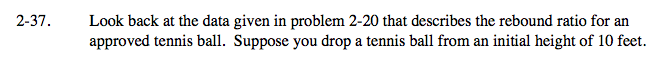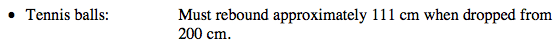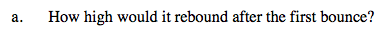Find the rebound ratio by dividing the rebound by the initial height.

$\frac{111}{200}=0.555$

Multiply the new initial height by the ratio.

10 · 0.555

5.55 ft.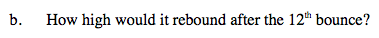Multiply the initial height by 0.555, twelve times.

10 · (0.555)12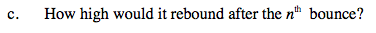Remember that the initial height is 10 feet and the ratio is 0.555.